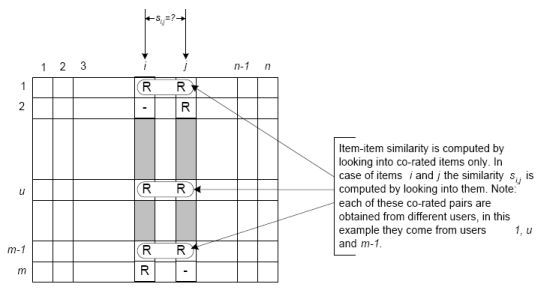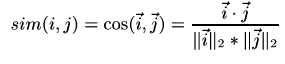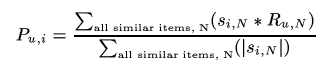# Item-based collaborative filtering

Item-based collaborative filtering is a model-based algorithm for making recommendations. In the algorithm, the similarities between different items in the dataset are calculated by using one of a number of similarity measures, and then these similarity values are used to predict ratings for user-item pairs not present in the dataset.

## Similarities between items

The similarity values between items are measured by observing all the users who have rated both the items. As shown in the diagram below, the similarity between two items is dependent upon the ratings given to the items by users who have rated both of them:## Similarity measures

There are a number of different mathematical formulations that can be used to calculate the similarity between two items. As can be seen in the formulae below, each formula includes terms summed over the set of common users U.

### Cosine-based similarity

Also known as vector-based similarity, this formulation views two items and their ratings as vectors, and defines the similarity between them as the angle between these vectors:### Pearson (correlation)-based similarity

This similarity measure is based on how much the ratings by common users for a pair of items deviate from average ratings for those items:This similarity measurement is a modified form of vector-based similarity where we take into the fact that different users have different ratings schemes; in other words, some users might rate items highly in general, and others might give items lower ratings as a preference. To remove this drawback from vector-based similarity, we subtract average ratings for each user from each user's rating for the pair of items in question:## From model to predictions

Once we make a model using one of the similarity measures described above, we can predict the rating for any user-item pair by using the idea of weighted sum. First we take all the items similar to our target item, and from those similar items, we pick items which the active user has rated. We weight the user's rating for each of these items by the similarity between that and the target item. Finally, we scale the prediction by the sum of similarities to get a reasonable value for the predicted rating:## Our implementation

We implemented item-based collaborative filtering using these parameters:

• Minimum number of users for each item-item pair: 5 (see below for explanation)
• Number of similar items stored: 50

## Challenges

We tried item-based collaborative filtering on the Movielens dataset, but as the Results page shows, it didn't perform very well in testing. In particular, we isolated two main problems, which were mainly due to the sparsity of the data:

• The first problem manifested itself during adjusted-cosine similarity measurement calculation, in the case when there was only one common user between movies. Since we subtract the average rating for the user, the adjusted-cosine similarity for items with only one common user is 1, which is the highest possible value. As a result, for such items, which are common in the Movielens database, the most similar items end up being only these items with one common user. The solution we implemented was to specify a minimum number of users (in this case, 5) that two movies needed to have in common before they could be called similar.
• The second challenge arose when we used weighted sum to calulate the rating for test user-movie pairs. Since we were storing only 50 similar movies for each movie, and for each target movie, we only consider the similar movies that the active user has seen, it was often the case with the Movielens dataset that there weren't many such movies for many of the users. This resulted in bad predictions overall for large test sets. Because this was due to the sparsity of the dataset itself, we couldn't come up with a straightforward solution to this problem.

## References

 M.Deshpande and G. Karypis. Item-based top-n recommendation algorithms. ACM Trans. Inf. Syst., 22(1):143-177, 2004.

 B.M. Sarwar, G. Karypis, J.A. Konstan, and J. Reidl. Item-based collaborative filtering recommendation algorithms. In Proceedings of the 10th International World Wide Web Conference, pages 285-295, 2001.